# 共轭传热

2014年 1月 6日

### 固体和流体传热

#### 固体中的传热

\rho C_{p} \frac{\partial T}{\partial t}=\nabla \cdot (k\nabla T) +Q

#### 流体中的传热

1. 流体传递意味着能量传递，在传热方程中，该项作为对流贡献。根据流体和流型的热学属性，可能以对流或传导传热为主。
2. 流体流动的粘性效应将导致流体加热。通常忽略该项，但在快速流动的粘性流体中，则应考虑。
3. 如果流体密度会根据温度变化，则应在传热方程中加入压力功贡献项。它代表了诸如压缩空气会产生热之类的著名效应。

\rho C_{p} \frac{\partial T}{\partial t}+\rho C_p\bold{u}\cdot\nabla T= \alpha_p {T}\left( \frac{\partial p_\mathrm{A}}{\partial t}+\bold{u}\cdot\nabla p_\mathrm{A}\right)+\tau : S+\nabla \cdot (k\nabla T) +Q

### 共轭传热应用

#### 有效传热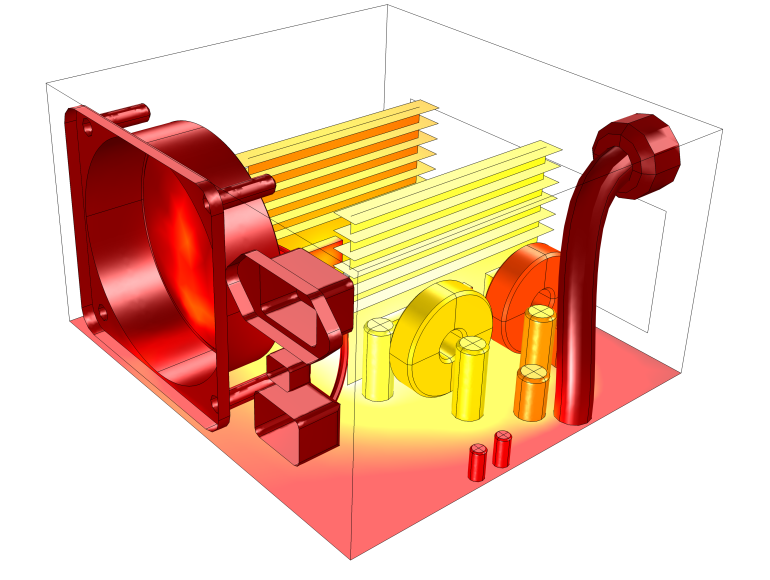#### 节能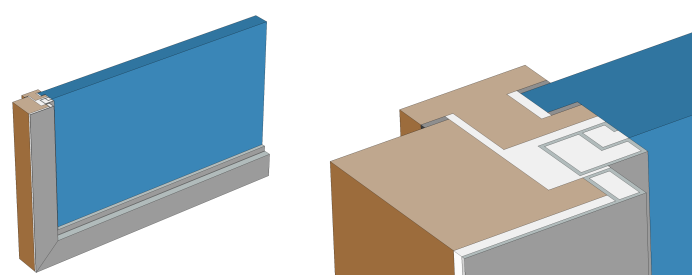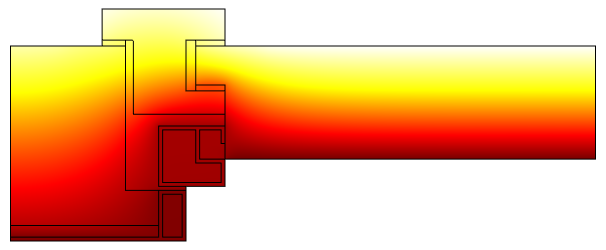ISO 10077-2:2012 标准的窗框和玻璃横截面的温度剖面图 (窗户的热性能)

### 流固耦合

#### 流体/固体界面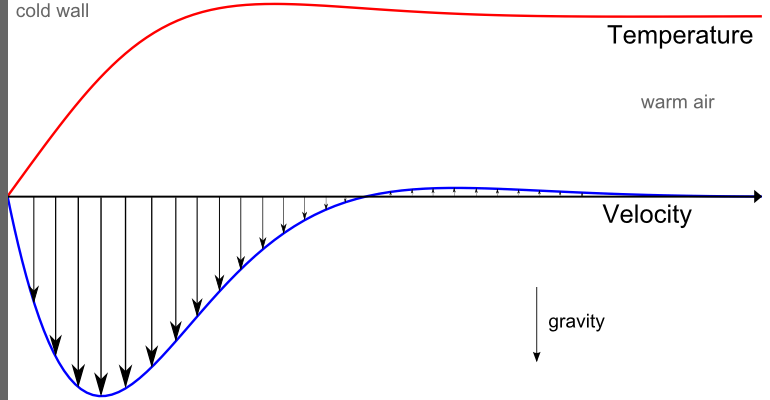#### 自然对流

Rayleigh 数 Ra 用于表征由自然对流带来的流型和传热。Rayleigh 数由流体材料属性、空腔尺寸 L ，以及温差 \Delta T定义，而温度差则根据流体周围的固体来设定：

Ra=\frac{\rho^2g\alpha_p C_p}{\mu k}\Delta T L^3

Grashof 数是另一个流型指标，它是浮力相对粘性力的比例：

Gr=\frac{\rho^2g\alpha_p}{\mu^2}\Delta T L^3

Rayleigh 数可通过 Prandtl 和 Grashof 数的关系式表示：Ra=Pr Gr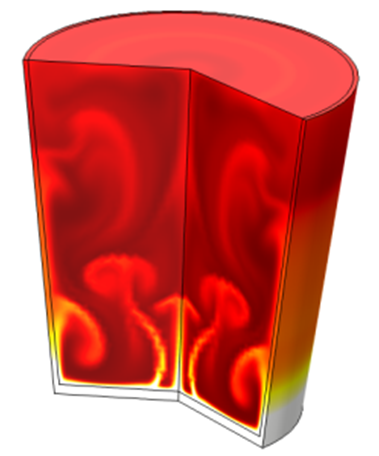#### 强制对流### 辐射传热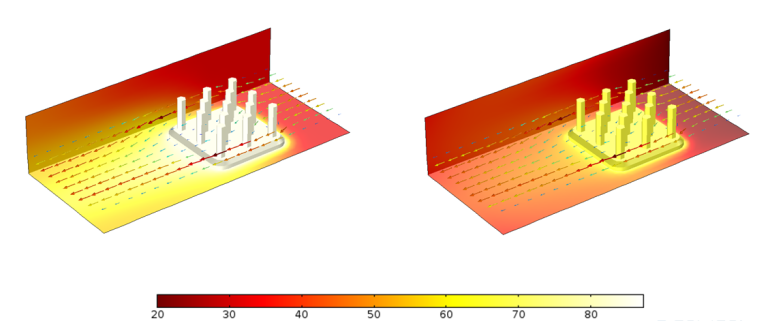### 标记

C_{p}：定压热容（SI 单位：J/kg/K）

g： 重力加速度（SI 单位：m/s2

Gr：Grashof 数（无量纲数）

k：导热系数（SI 单位：W/m/K）

L: 特征尺寸（SI 单位：m）

n：折射率（无量纲数）

p_\mathrm{A}：绝对压力（SI 单位：Pa）

Pr：Prandtl 数（无量纲数）

q：热通量（SI 单位：W/m2

Q：热源（SI 单位：W/m3

Ra：Rayleigh 数（无量纲数）

S：应变率张量（SI 单位：1/s）

T：温度场（SI 单位：K）

T_\mathrm{amb}：环境温度（SI 单位：K）

\bold{u}：速度场（SI 单位：m/s）

U:典型速度大小（SI 单位：m/s）

\alpha_{p}:热膨胀系数（SI 单位：1/K）

\delta_\mathrm{M}:动量边界层厚度（SI 单位：m）

\delta_\mathrm{T}：热层厚度（SI 单位：m）

\Delta T：特征温度差（SI 单位：K）

\varepsilon：表面发射率（无量纲数）

\rho：密度（SI 单位：kg/m3

\sigma：Stefan-Boltzmann 常数（SI 单位：W/m2T4

\tau：粘性应力张量（SI 单位：N/m2

#### 评论 (1)

##### 留言2020-07-03